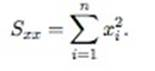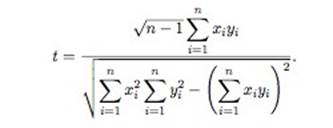# We Helped With This Statistics with R Programming Homework: Have A Similar One?

SOLVEDCategory Programming R | R Studio Graduate Solved Assignment Statistics

## Assignment Description

1. This problem is a follow up on problem 1 of assignment 5 about the regression through the origin model of the form

Y =β1x+ε, where ε is the standard normal distribution.

.      (a)  First, generate a predictor x as a random vector of length n = 100 from the standard normal and a response y as follows. y = 2x + ε

where ε is also a random vector of length n = 100 from the standard normal

that takes exactly the same values as x.

.            (b)  Perform a simple linear regression of y onto x, without an intercept.

Report the coefficient estimate βˆ1, the standard error of this coefficient estimate, and the t-statistic and p-value associated with the null hypothesis H0 : β1 = 0. Comment on these results. (You can perform regression without an intercept using the command lm(y x + 0).)

.      (c)  Now perform a simple linear regression of x onto y without an intercept. Report the coefficient estimate βˆ1, the standard error of this coefficient estimate, and the t-statistic and p-value associated with the null hypothesis H0 : β1 = 0. Comment on these results.

.      (d)  What are the relationships between (a), the results obtained in (b) and the ones in (c)?

.           (e)  For the regression of Y onto X without an intercept, the t-statistic for

H0 :β1 = 0 takes the form

T= B1 − β1 / S/ √ Sxx,

whereS2= S S E / n-1    and

Note that these formulas are slightly different from those given in (slide 29, LinearRegression-1), since here we are performing regression without an in- tercept.

(f) Show algebraically (to receive bonus points), and confirm numerically in R, that the t-statistic above can be written as (values).         (g)  Using the results from (d), argue that the t-statistic for the regression of y onto

x is the same as the t-statistic for the regression of x onto y.

.      (h)  In R, show that when regression is performed with an intercept, the tstatistic forH0 1 =0 is the same for the regression of y onto x as it is for the regression of x onto y.4. The data set low bwt.txt contains information for a sample of 100 low birth weight infants. The variables are

sbp : maternal systolic blood pressuresex : gender of the baby

toxemia : toxemia during pregnancy (yes or no) germ.hem : germinal matrix hemorrhage (yes or no) gest.age : gestational age in weeksapgar5 : five-minute APGAR score

.      (a)  Using germinal matrix hemorrhage as the response, fit a logistic regression model where the predictor variable x1 is the 5-minute APGAR score. Write the equation and interpret β1, the estimated coefficient of

Apgar score.

.         (b)  What is the estimate and 95% confidence interval for the slope

(coefficient for apgar5) in the odds ratio scale? Interpret the estimate

(what does the odds ratio mean?).

.      (c)  At the 0.05 level of significance, test the null hypothesis: H0 : β1 = 0 where β1 is the coefficient for apgar5.

.      (d)  If a new infant from the population has an APGAR score of 3 what is the predicted probability that this child will experience a brain hemorrhage? What is the probability if the child’s score is 7?

Is it free to get my assignment evaluated?

Yes. No hidden fees. You pay for the solution only, and all the explanations about how to run it are included in the price. It takes up to 24 hours to get a quote from an expert. In some cases, we can help you faster if an expert is available, but you should always order in advance to avoid the risks. You can place a new order here.

How much does it cost?

The cost depends on many factors: how far away the deadline is, how hard/big the task is, if it is code only or a report, etc. We try to give rough estimates here, but it is just for orientation (in USD):

 Regular homework \$20 - \$150 Advanced homework \$100 - \$300 Group project or a report \$200 - \$500 Mid-term or final project \$200 - \$800 Live exam help \$100 - \$300 Full thesis \$1000 - \$3000

How do I pay?

Credit card or PayPal. You don't need to create/have a Payal account in order to pay by a credit card. Paypal offers you "buyer's protection" in case of any issues.

Why do I need to pay in advance?

We have no way to request money after we send you the solution. PayPal works as a middleman, which protects you in case of any disputes, so you should feel safe paying using PayPal.

Do you do essays?

No, unless it is a data analysis essay or report. This is because essays are very personal and it is easy to see when they are written by another person. This is not the case with math and programming.

Why there are no discounts?

It is because we don't want to lie - in such services no discount can be set in advance because we set the price knowing that there is a discount. For example, if we wanted to ask for \$100, we could tell that the price is \$200 and because you are special, we can do a 50% discount. It is the way all scam websites operate. We set honest prices instead, so there is no need for fake discounts.

Do you do live tutoring?

No, it is simply not how we operate. How often do you meet a great programmer who is also a great speaker? Rarely. It is why we encourage our experts to write down explanations instead of having a live call. It is often enough to get you started - analyzing and running the solutions is a big part of learning.

What happens if I am not satisfied with the solution?

Another expert will review the task, and if your claim is reasonable - we refund the payment and often block the freelancer from our platform. Because we are so harsh with our experts - the ones working with us are very trustworthy to deliver high-quality assignment solutions on time.

Customer Feedback

"Thanks for explanations after the assignment was already completed... Emily is such a nice tutor! "

Order #13073